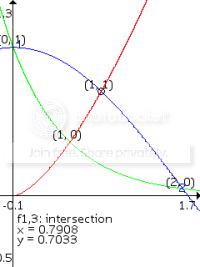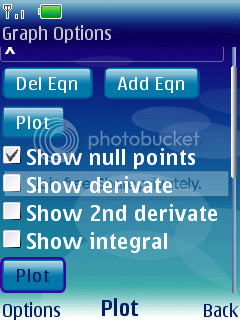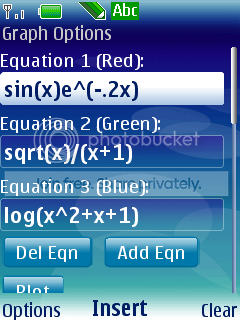Home / Java / GraphViewer v1.0.5 Java (Jar/JAD)GraphViewer is a graphical calculator for your mobile phone. With GraphViewer you can plot equations using the build-in functions, calculate its derivate, show a table with the functions critical points and even many more features.### Features

• You can enter any mathematical function you want to plot and evaluate
• You can zoom in/out and move the canvas infinitely
• The solutions (null points) of an equation can be calculated and shown
• Easy function input with the insert function command (list of commands)
• Multiple functions can be plotted in different colors at once (see screenshots)
• The first and second derivate can be shown
• You can calculate the local minima and maxima of the function
• You can calculate the function inflection points
• Show the integral of a function (the constant term will be chosen, so that the integral goes through the origin)
• The intersection points of the different equations are calculated and can be shown
• You can interactively evaluate the functions at their critical points
• You can show a function table with the function coordinates at its critical points (So the null points, minima, maxima, inflection points… are automatically calculated)
• Build-in samples show the possibilities of all functions
• Calculate any function point up to 14 decimals.
• In the settings you can set the internal precision, so you can choose between low precision (fast) or high precision (slow), depending on the speed of your mobile. These settings are saved, so you don’t have to reset it each time.
• You can save your equations and add them to the samples library of the Graph Viewer. These samples are saved persistent, for as long as you want.